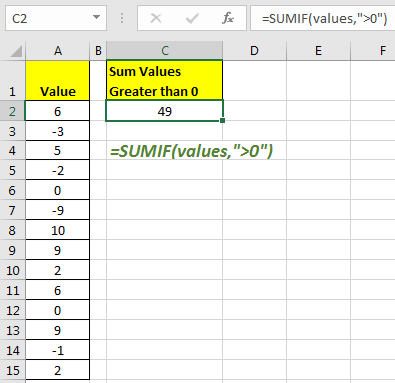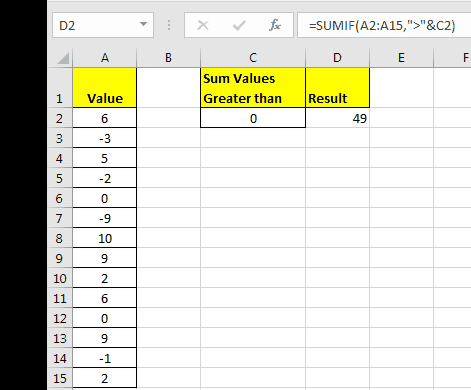# How to Sum If Greater Than 0 in Excel

There are times, when we have data that have negative value and positive values too. Now to sum only positive values, we need to sum values that are greater than 0. To do so, we will use SUMIF function of Excel.

Generic Formula:

=SUMIF(range,”>0”)

Range: the range that you want to sum.
“>0”: Signifies that, you want to sum values that are greater than 0.

Example 1:

Here we have a range named values. Now, in this range i want to sum values that are greater than zero. To do so. Right this formula in any cell.

=SUMIF(values,">0")

The SUM if function will return sum of values that are greater than zero.In this example, I have named range A2:A15 as values.
Now, if you want to get sum of values that are greater than from a given value in another cell than you can use below technique.

Sum If Greater Than Value in Another Cell
In above example we used hard coding to get sum of values that are greater than 0. But many times you would want it to be check value from another cell. We have a generic formula for that too.

Generic Formula:

=SUMIF(range,”>”&cell_reference)

Cell_reference: the reference of cell that contains the value that you want to check.

Example 2:

In this example, I have same range for sum. The only difference is that I want to sum if greater than value in cell C2.

To achieve this write below formula.

=SUMIF(A2:A15,">"&C2)Similarly if, you want to check any condition you can have that in that in “”. For example, (“>”,”<”,”<>”,”>=”,”<=”). SUMIF function supports all these functions.

Important: To sum if a value is equal to sum value, you don’t need to use “”. Just pass that value or reference of that value.

This was simple. We have many other important formulas and techniques to simplify your work and increase productivity.

Popular Articles

50 Excel Shortcut to Increase Your Productivity : Get faster at your task. These 50 shortcuts will make you work even faster on Excel.

How to use the VLOOKUP Function in Excel : This is one of the most used and popular functions of excel that is used to lookup value from different ranges and sheets.

How to use the COUNTIF function in Excel : Count values with conditions using this amazing function. You don't need to filter your data to count specific values. Countif function is essential to prepare your dashboard.

How to use the SUMIF Function in Excel : This is another dashboard essential function. This helps you sum up values on specific conditions.

Terms and Conditions of use

The applications/code on this site are distributed as is and without warranties or liability. In no event shall the owner of the copyrights, or the authors of the applications/code be liable for any loss of profit, any problems or any damage resulting from the use or evaluation of the applications/code.